• MATLAB矩阵运算中，往往需要一种快速找到最大值及其坐标的方法。
• 摘要：做信号处理运算时，很多时候都需要找到最大值所在的位置。在matlab里面一条指令就行了，但是在keil下，就需要自己写算法了。本文就用几行代码，教您找到最大值下标（角标）。
摘要：做信号处理运算时，很多时候都需要找到最大值所在的位置。在matlab里面一条指令就行了，但是在keil下，就需要自己写算法了。本文就用几行代码，教您找到最大值下标（角标）。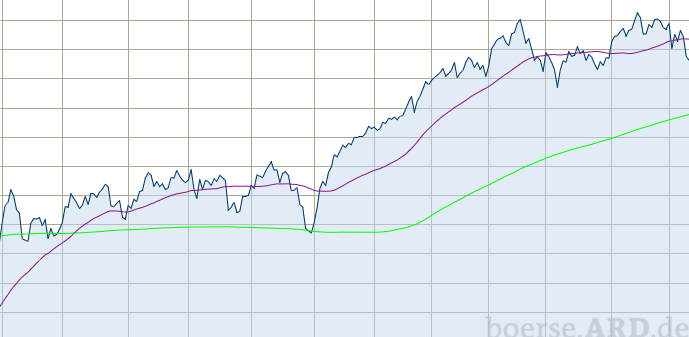如下图，想找到最大值所在位置，怎么办？matlab下，找到最大值仅需要一个语句。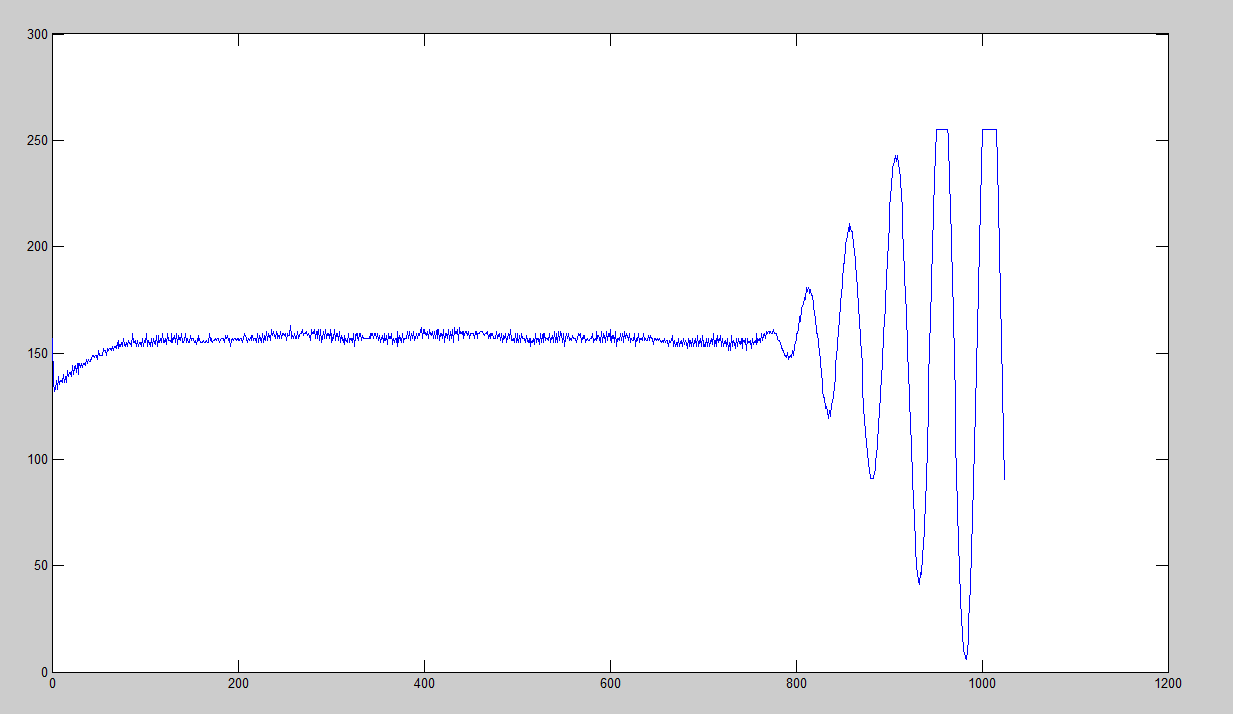1.matlab下怎么找最大值角标
输入语句[m,index]=max(q1)就能求出来最大值m是多少，它的位置index是多少。
[m,index]=max(q1)
结果如下图所示，这个例子中有一个特殊之处，就是最大值所处的位置有好几个，matlab找到的最大值位置是第一次出现的时候。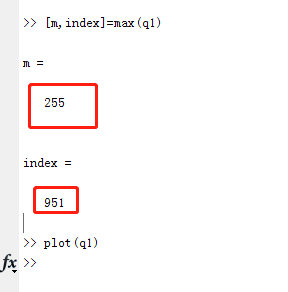2.keil下怎么找到最大值角标
keil下找最大值角标的思路和冒泡排序找最大值的思路是相同的，就是用一个临时变量（这里假设为max），让这个临时变量与数组中的每一个数据比较大小，如果临时变量小于这个数组数据，那么就让临时变量的数据更新为当前的这个数组数据，并记录下来它的角标，用index_max来保存下来。如果这个数据一直比到最后都没有找到比他大的数，那么它就是那个最大的数了，index_max也一直保留着最后的状态。它就是您要找的最大值角标了。
max = test_array;

for(i=0;i<FFT_LENGTH;i++)
{
if (max < test_array[i])
{
max = test_array[i];
index_max = i;
}
}
printf("%d\r\n",index_max);	
这个程序非常简单，但是经常被用到。
展开全文keil 下标
• 大多数算术运算传播 NaN ，但 max 和 min 忽略它们。 例如 3+NaN 和 sum([3 NaN]) 返回 NaN，但 max(3, NaN) 和 max([3 NaN]) 返回 3。 有时， max 和 min 的行为与其他算术函数类似是很有用的。 例如，如果您...
• 本代码主要利用MATLAB工具实现MATLAB——多项式求导运算，简单明了，易于理解
• ## MATLAB教程（1） MATLAB 基础知识

万次阅读 多人点赞 2017-10-26 20:57:32
去年看过一点点MATLAB，很久不用，遗忘惊人。为了加深自己的印象，扎实基础，现将官网上的基础教程做简单的翻译。 首先，以下从九个部分简单介绍基础入门知识。第一部分：MATLAB显示桌面的基本布局...

初学。去年看过一点点MATLAB，很久不用，遗忘惊人。为了加深自己的印象，扎实基础，现将官网上的基础教程做简单的翻译。

首先，以下从九个部分简单介绍基础入门知识。
第一部分：MATLAB显示桌面的基本布局
https://cn.mathworks.com/help/matlab/learn_matlab/desktop.html
1.启动MATLAB后，桌面会显示默认布局，主要有以下三个部分：
当前文件夹命令行窗口工作空间
2.在MATLAB中一般需要创建变量和调用函数。
直接在命令行中输入变量名以及其值即可（比如：a = 1）,多个变量的创建同理，不再赘述。
需要注意的是，如果没有定义输出变量，MATLAB会使用一个名为ans的变量来储存计算结果。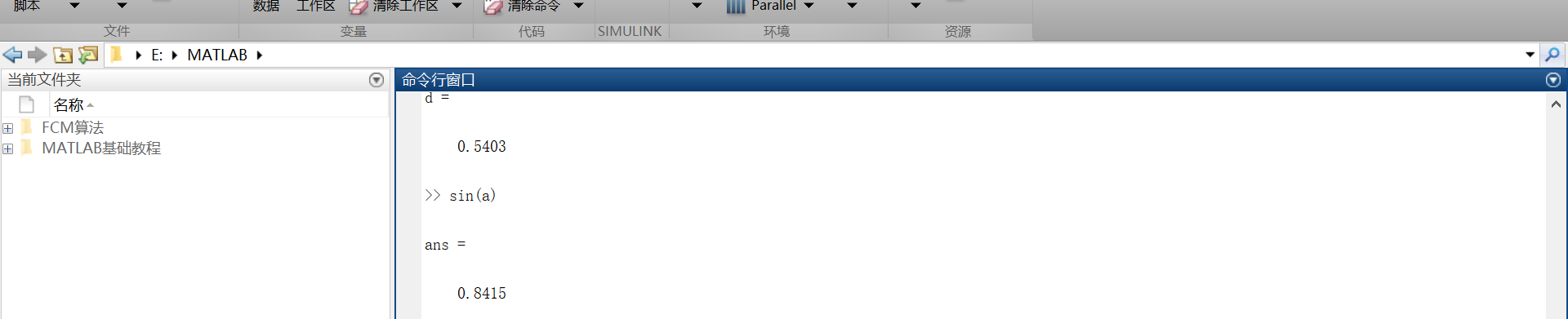3.如果语句以分号结束，表示MATLAB内部会执行这一运算，但是命令行不对计算结果进行输出。
第二部分：矩阵和数组
https://cn.mathworks.com/help/matlab/learn_matlab/matrices-and-arrays.html
大多数的编程语言一次性只能对一个数进行操作，而MATLAB主要被用来对整个矩阵或者数组进行操作。不管是什么类型的数据，所有的额MATLAB变量都是数组。矩阵是常用于线性代数的二维数组。
1.数组的创建
在一个单行中创建一个拥有四个元素的数组，其各个元素之间用逗号或者空格隔开。  例如：  a = [1 2 3 4]  这种数组叫做单行矩阵（行向量）。创建一个多行矩阵，其各个行之间用分号隔开。  例如：  b = [1,2;3,4;5,6]还可以用函数来创建矩阵。  例如：创建一个5行1列每一个元素都是0的列向量。  z = zero(5,1)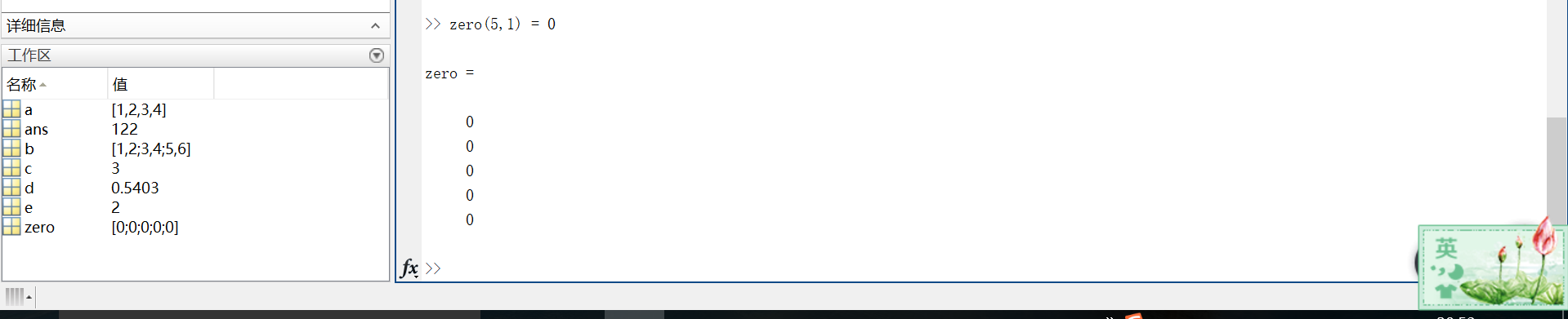2.矩阵和数组的操作
在MATLAB中，允许用一个单一的运算或者函数对矩阵中所有的数据进行处理（操作）。
例如：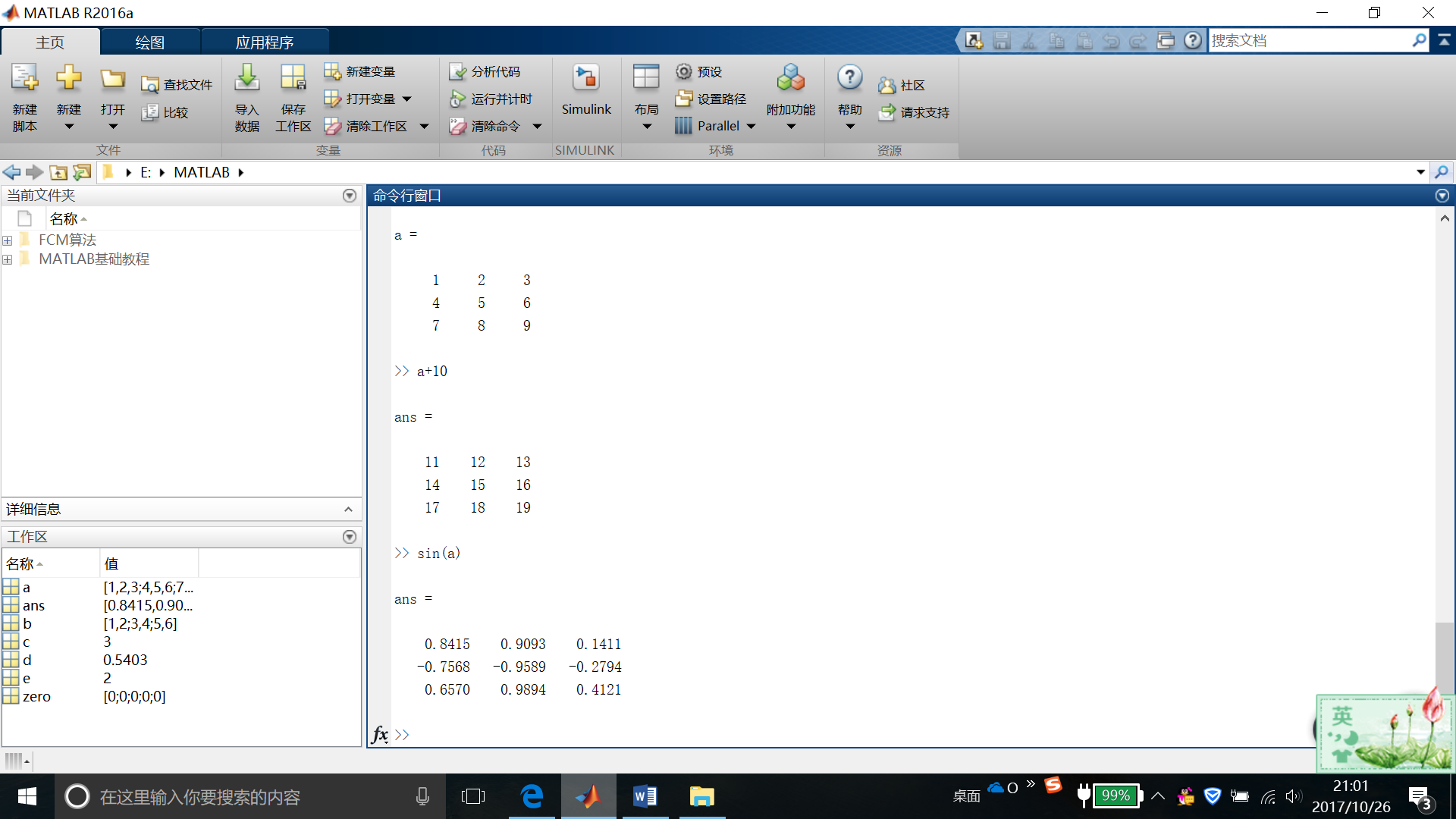如果要对矩阵进行转置，则使用单引号(‘)  例如：a’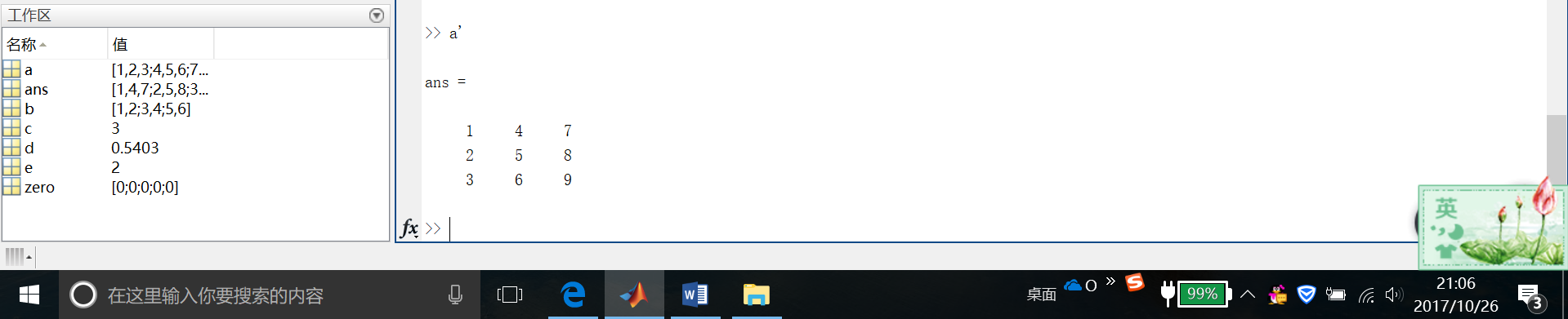可以通过执行标准矩阵乘法，通过*运算符来计算内积。
这是我自己的结果截图……很明显是不对滴~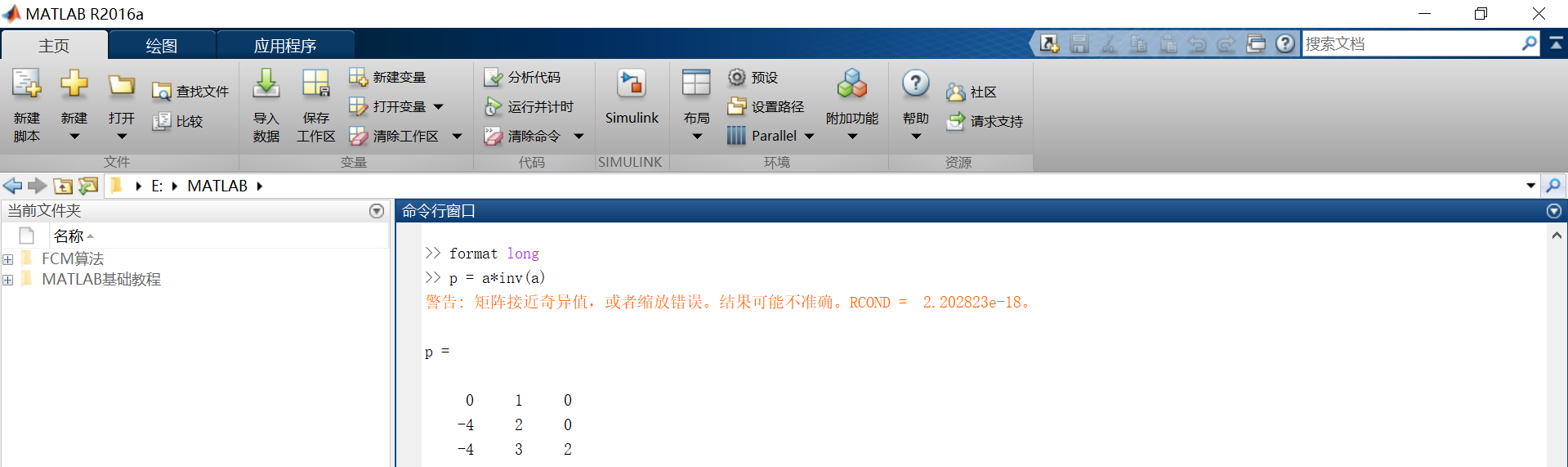然后下面是官网爸爸给的运行结果。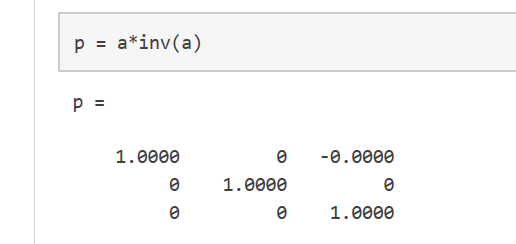我们都知道，一个矩阵与它的逆矩阵相乘的结果的确应该等于单位矩阵。至于这里为什么不对，还希望各位大佬们能不吝赐教，大恩不言谢~
这里要注意的是，p不是一个整数值矩阵，MATLAB存储数字是以浮点型存储的，真实值和它的浮点数在运算时有小小的不同，我们可以使用格式化命令，显示更多的小数位数。

比如：  format long  p = a*inv(a)

可以使用format short来重置到较短格式的使用。
x = a.*a用来表示对矩阵的每一个元素做平方操作。  若需要对每一个元素做立方运算，则用x = a.^3(如图)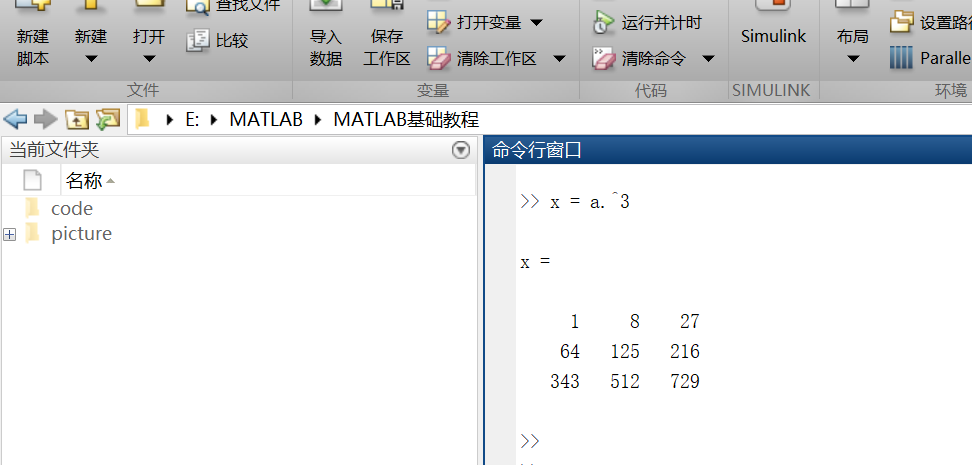矩阵的扩充  行方向上：A = [a,a]  列方向上：A = [a; a]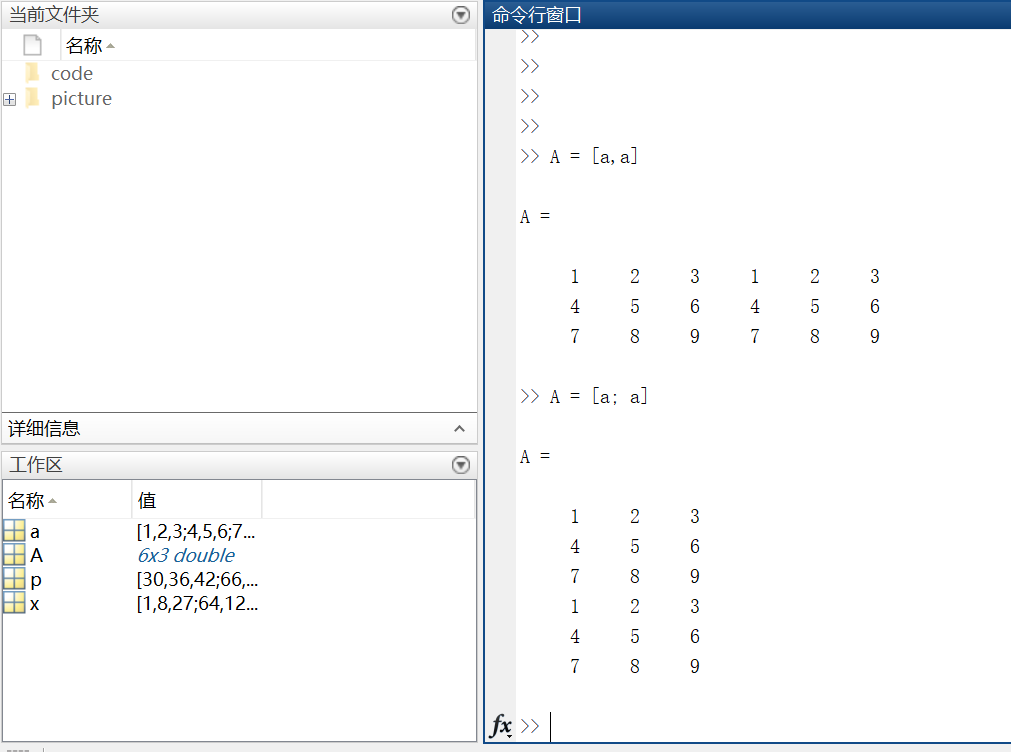复数  复数由实部和虚部组成，它的虚部是-1的平方根。  即sqrt(-1) = 0.0000 + 1.0000i  一般用i或者j来代表复数的虚部部分。
第三部分：数组的索引
https://cn.mathworks.com/help/matlab/learn_matlab/array-indexing.html
MATLAB中的每一个变量都是可以存储多个值的数组。如果想要使用数组中的某一个值，则需要用到索引。
1.单个元素的索引
从一个数组中索引某个元素一般有两种方法：  第一种比较常见：数组名加要索引的元素所在的行和列，例如A(2，4) = 8。  第二种不太常用：数组名加一个下标，该下标按列遍历。A(14) = 8。【这里不太好表达，上图吧~】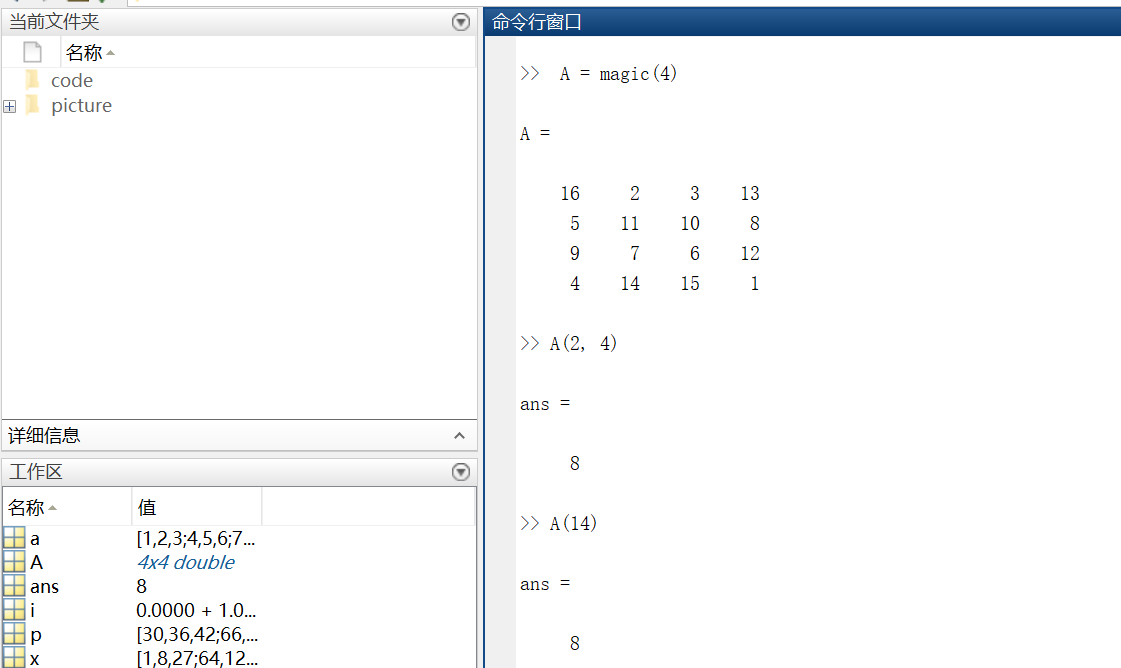再多说一句，其实A(14)中的14表示按列数第14个元素！  利用下标索引元素这种方式叫做线性索引。  注意：如果索引超出矩阵维度，MATLAB会报错。
2.多个元素的索引  引用数组的多个元素，使用冒号操作符m : n(m，n分别表示索引开始和结束的范围)
例1索引第二列第1个元素到第3个元素。  例2索引第三行全部元素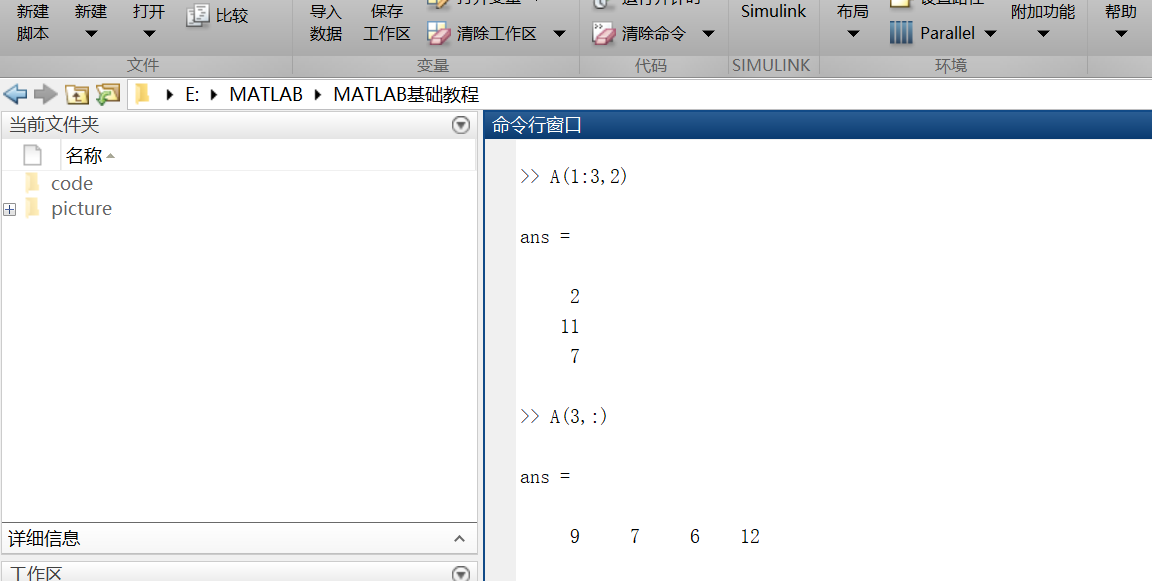不难发现，索引行则将行数放在逗号前；索引列要将列数放在逗号后。
另外，冒号运算符也可以帮助创建一组等距的矢量值，例如：  B = 0:10:100  —->B = 0 10 20 30 40 50 60 70 80 90 100  输出一个从0到100步长为10的数组。
第四部分：工作空间变量
https://cn.mathworks.com/help/matlab/learn_matlab/workspace.html
工作区包含你创建的变量或者从数据文件或其他程序导入到MATLAB中的变量。例如，我现在创建两个变量 ：  A = magic(4)  B = rand(3,5,2)
我们可以通过whos来看工作空间的内容。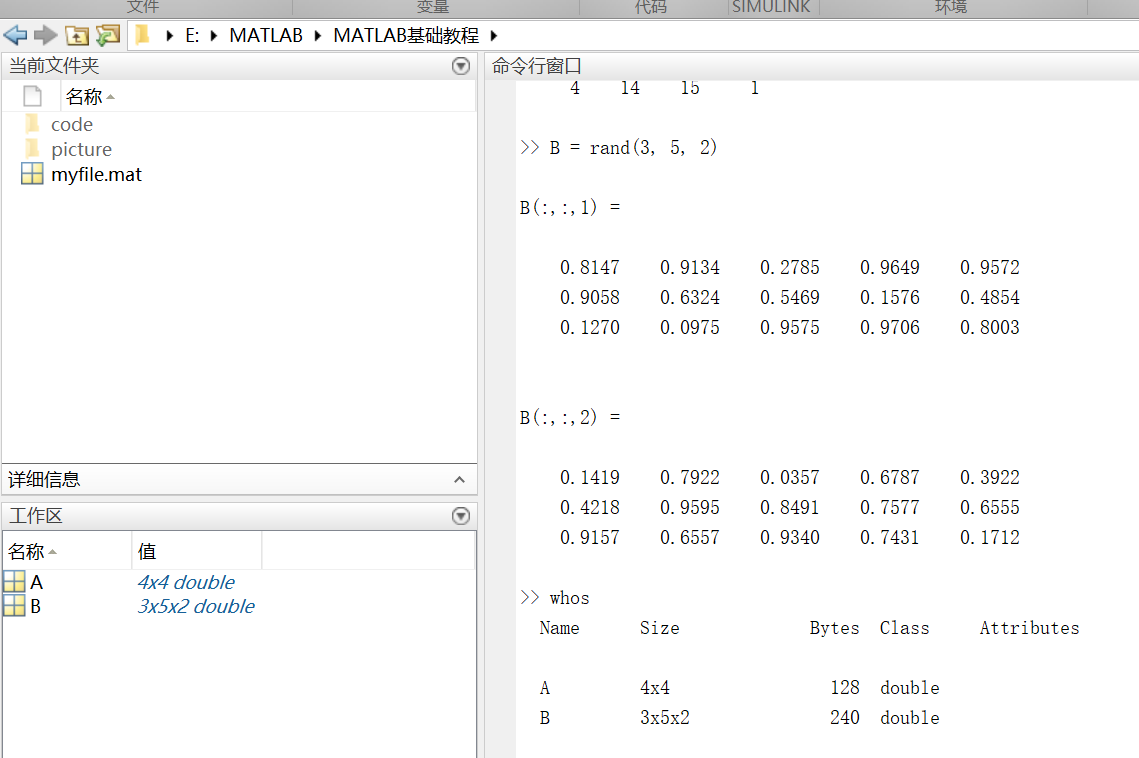第五部分：文本和字符
https://cn.mathworks.com/help/matlab/learn_matlab/character-strings.html
在处理文本时，将其中的字符序列用单引号括起来，可以将文本分配给变量。  例如：myText = ‘Hello, world’;如果文本本身包括一个单引号，则在定义时使用两个单引号。  例如：otherText = ‘You”re right’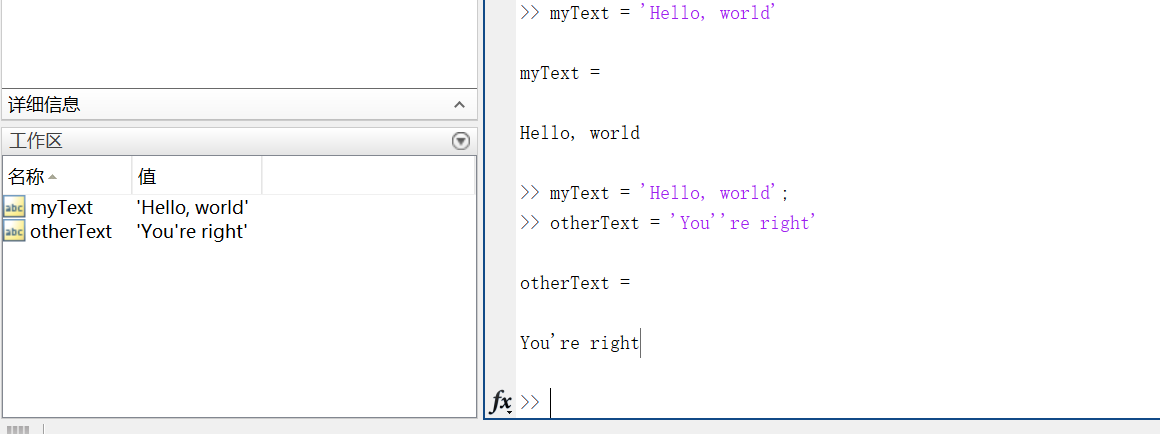同所有的MATLAB变量一样，上述创建的两个变量也都是数组，他们的数据类型是字符型。注意：字符数组也可以像数字数组那样用方括号括起来表示。
要将数字转换成字符，就要用到函数。例如num2str()或者int2str()。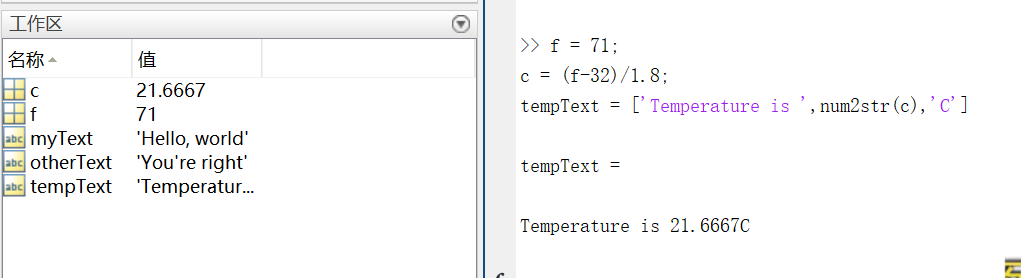第六部分：函数调用
https://cn.mathworks.com/help/matlab/learn_matlab/calling-functions.html
MATLAB提供了大量函数来执行计算任务，函数相当于其他编程语言中的子程序或方法。  例如求最大值的函数，max()。
将输入参数用括号括起来，如果有多个参数，则将参数用逗号隔开。  一般通过将函数值赋给某个变量这种方式来返回（输出）函数值，如果有多个输出参数，则需将它们用方括号括起来。  例如：  A = [1 3 5];  [maxA,location] = max(A)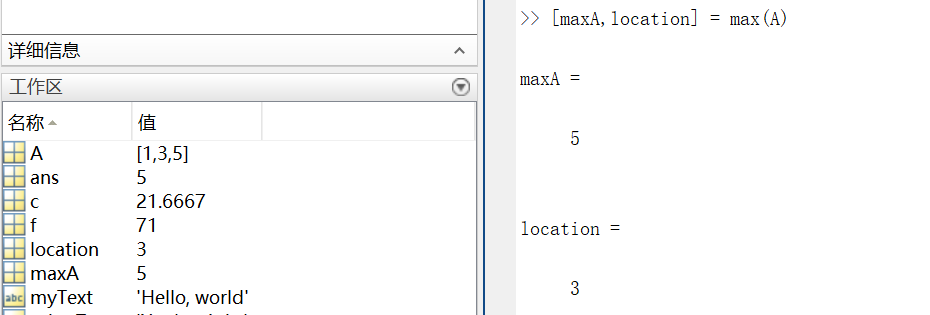可以用单引号括住任何字符进行输入。  例如：  disp(‘hello world’)  ===>输出 hello world

disp()用来显示变量的内容,括号里可以是字符,结构体,矩阵等等，类似于c语言的printf()。

clc函数被用来清空命令行。
第七部分：二、三维图
https://cn.mathworks.com/help/matlab/learn_matlab/plots.html
折线图 （1） 画图 x = 0:pi/1000:2*pi;  y = sin(x);  plot(x,y)
这里x就用到了前面说到的索引。x表示0到2*pi之间步长为pi/100的值。  二维图如下：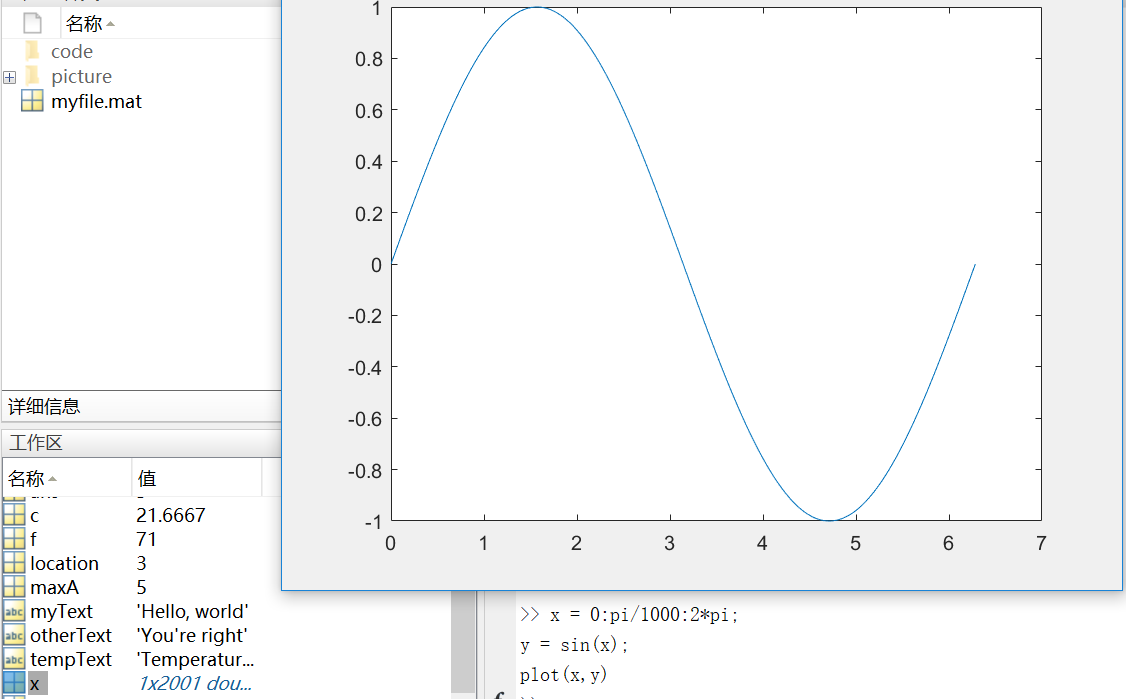这里多说一下步长，步长决定了横轴取值的密集程度。下图是将步长改为pi/10之后的图像。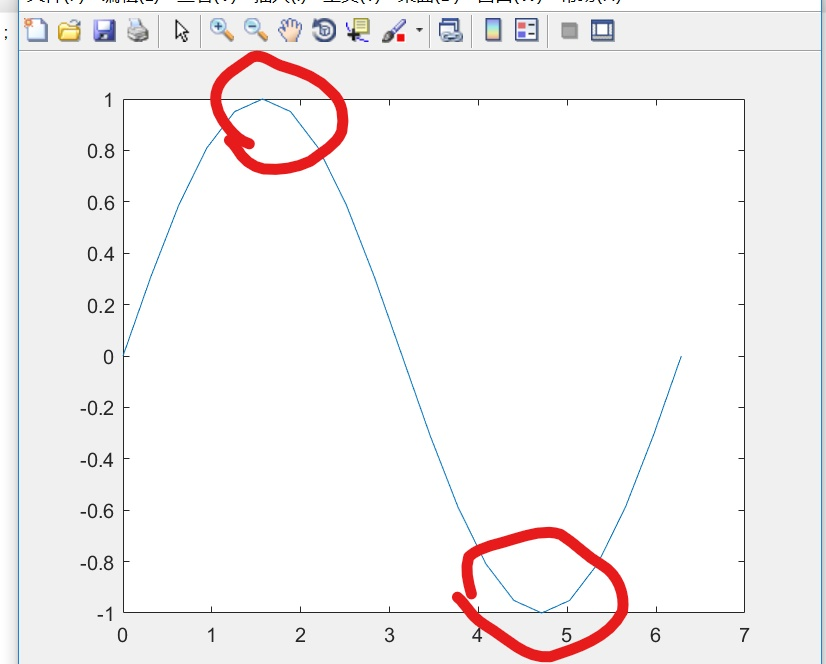对比图中标记处不难发现，步长增大，图像的精确度变低，所以这里尽量保证步长足够小，使得x可以取到足够多的值
（2） 坐标  我们可以对横轴和纵轴分别命名，并对上述正弦图像加上标题。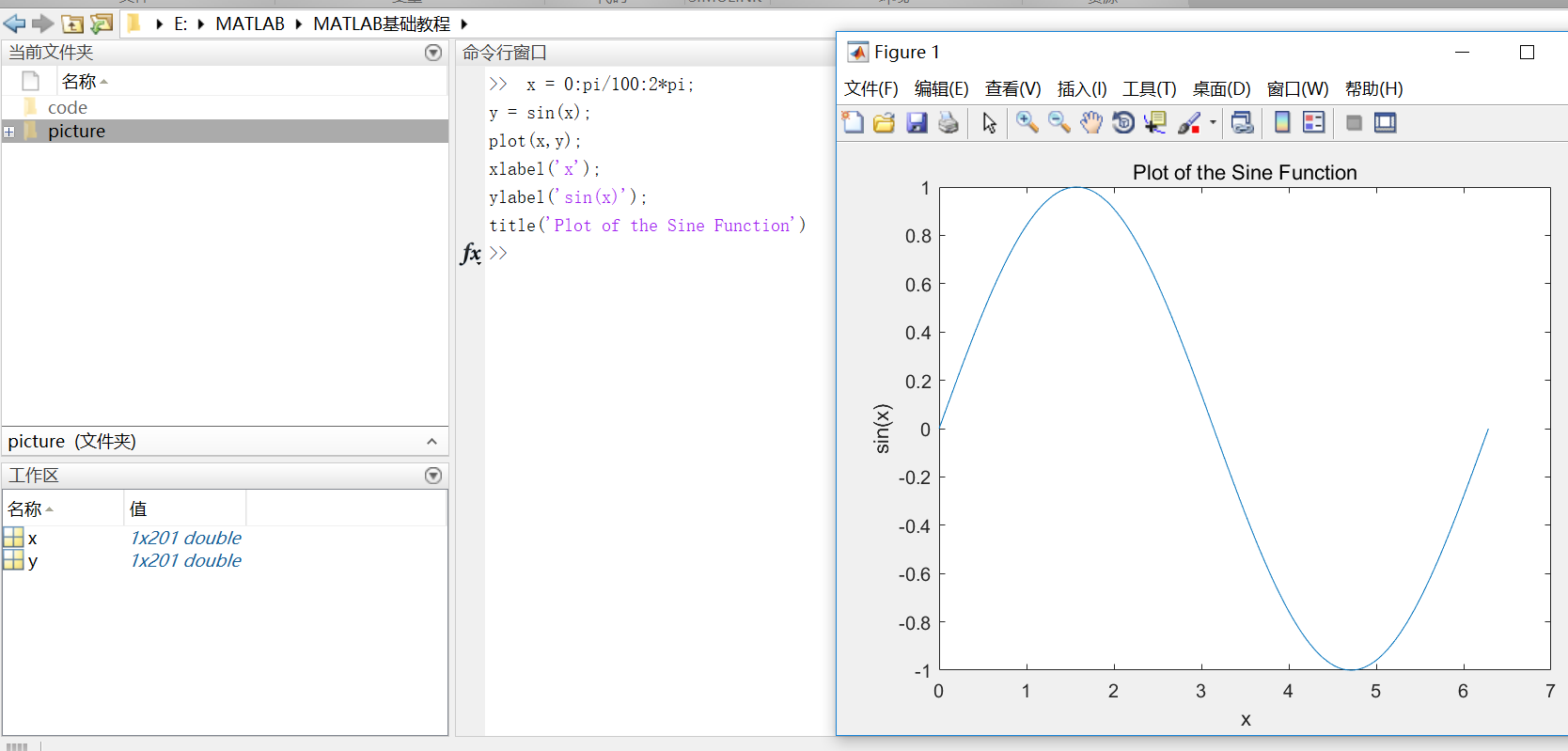（3）plot(x, y, ‘r–’)  通过在图像函数中添加第三个输入参数，可以用红色虚线绘制与原来相同的变量。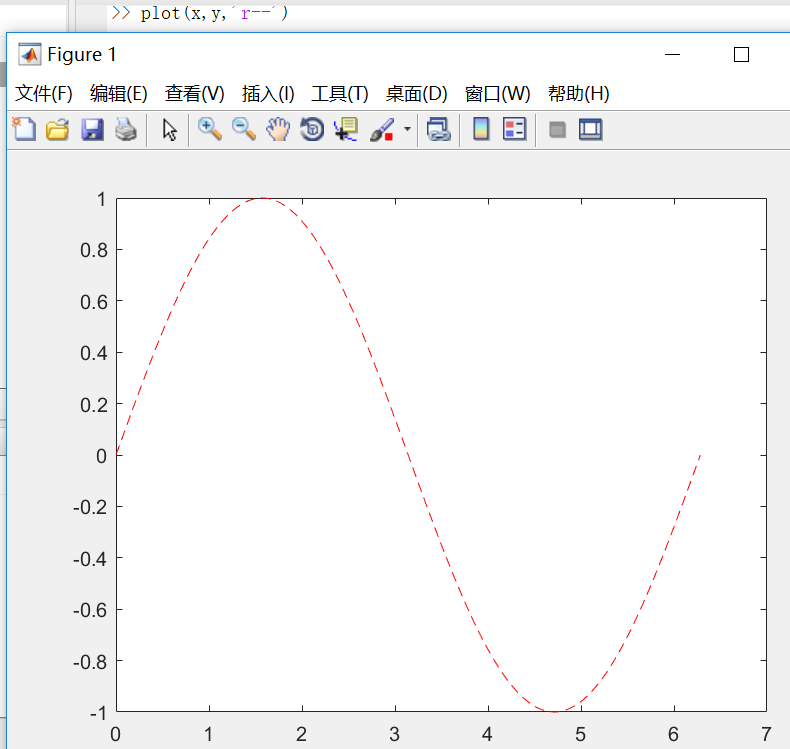其中第三个参数规定了线条的颜色、样式和标记的字符。（例如上例中的‘r–’表示红色虚线。）标记是指对每一个数据点用参数所给的标记形式进行表示。（例如’g:o’表示用‘o’连成一条绿色的线输出=.=我这个解释没谁了，讲不清楚还是上图吧…..）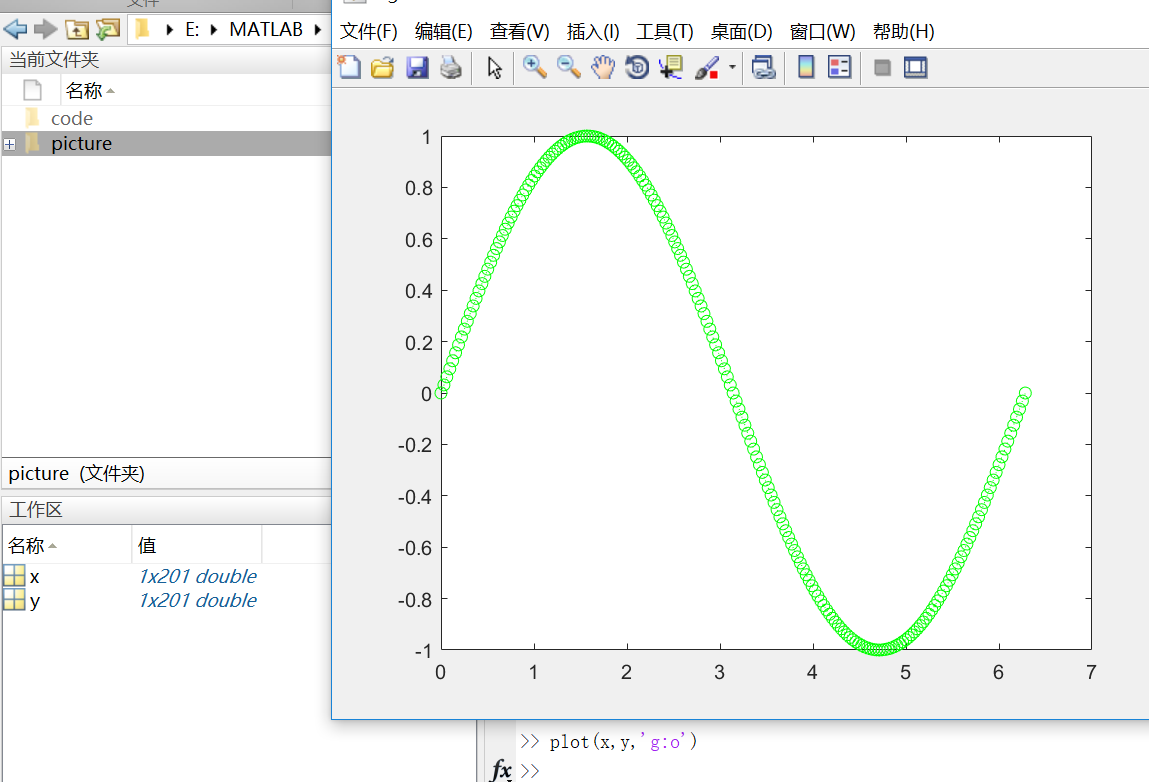这样的标记除了’o’，还有‘+’，‘*’等等。
这时候你会发现，我们开始为第一个图定义的标题和标签不再显示在当前图形窗口中了。这是因为在默认情况下，重新调用一个绘图函数，MATLAB就会清除数据，重置轴和其他元素来准备新的绘图。  若要将图添加到现有图形，使用hold命令即可。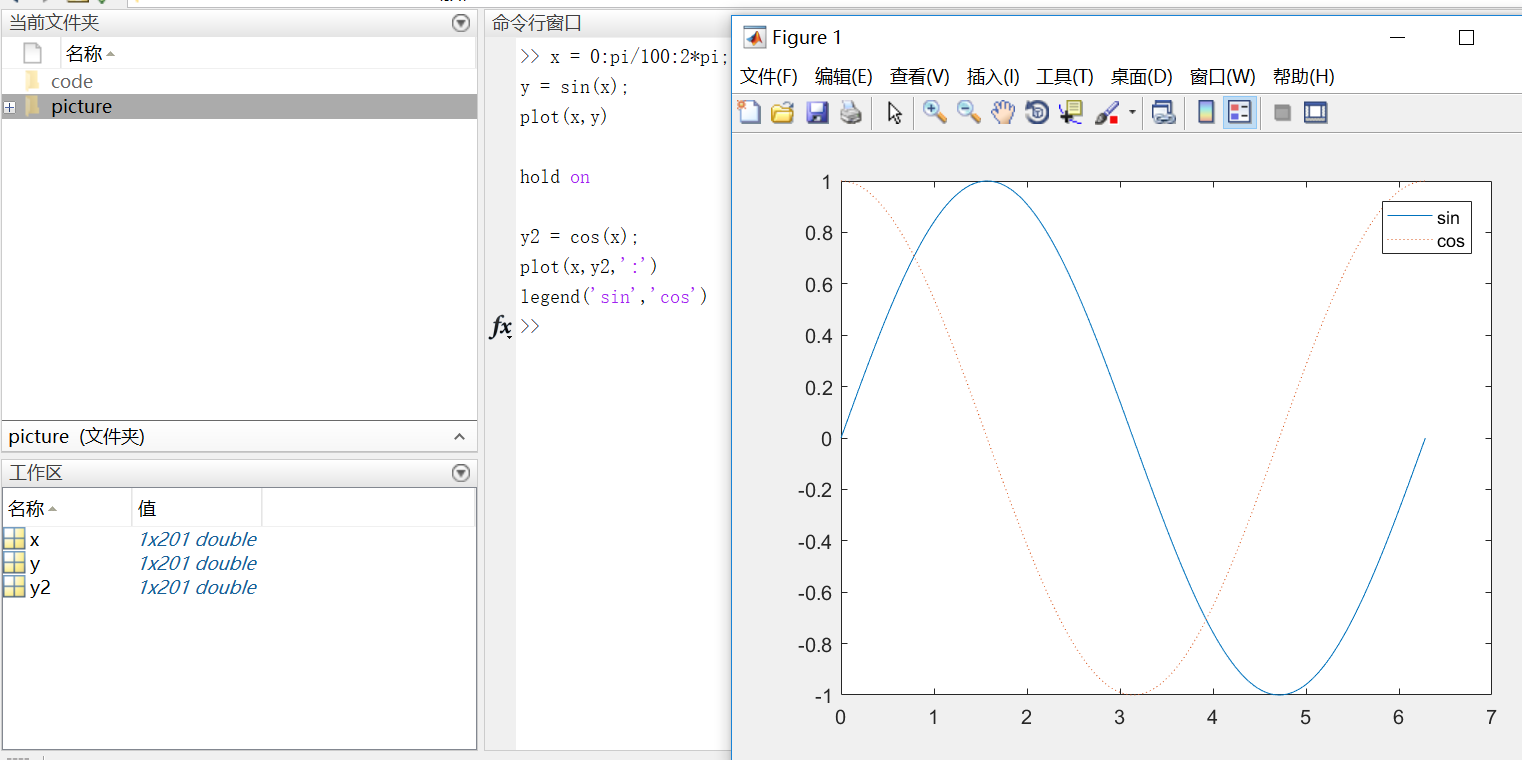在使用hold off或close the window命令之前，所有的图都会出现在当前的图形窗口中。
3D图像 3D图像通常用有两个参数的函数来定义一个曲面，z = f(x,y) 。  对于z，首先使用meshgrid命令在函数定义域内创建若干(x,y)。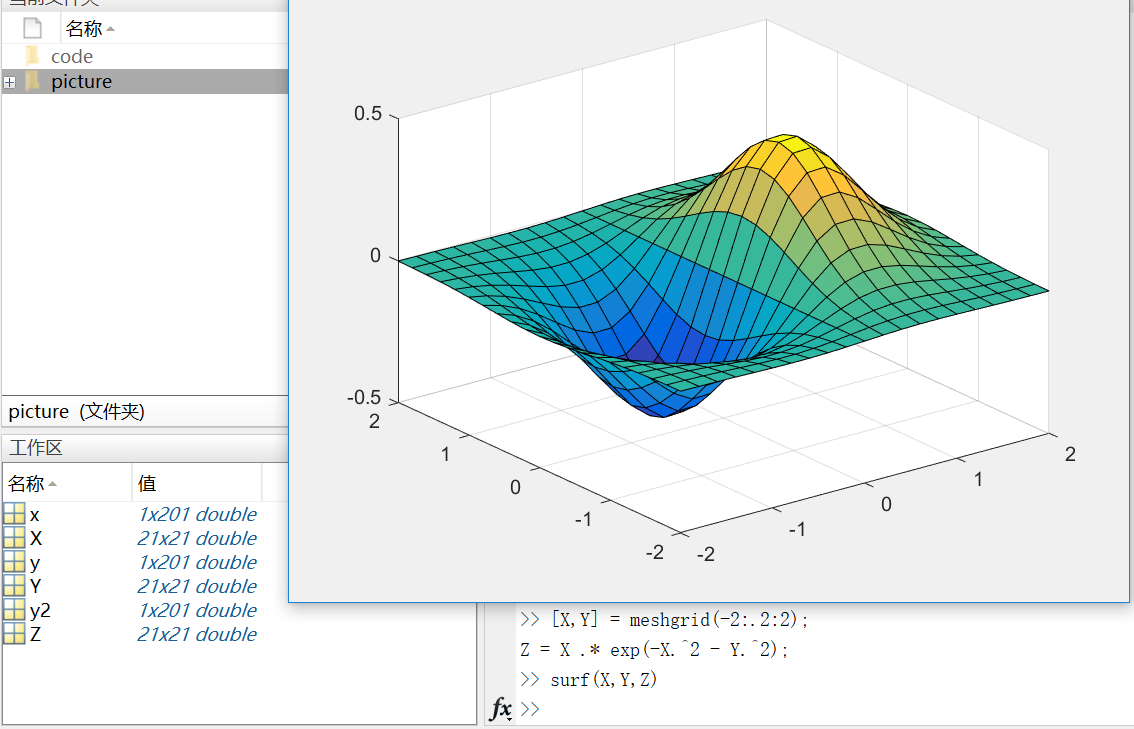这里需要注意的是，在matlab中，.2代表0.2  (-2:.2:2)表示在-2到2的区间上以0.2的长度为间隔的向量；
当变量x是由向量、数组或隔开的点组成（如x=0：0.1：1）时，则后面有x变量的方程要加点 (.)，例如：y=x.*exp(-x.*2)  但是如果x是连续的变量，如syms x，则方程写成y=x*exp(-x*2)。
Subplots  你可以用subplot函数在同一窗口的不同区域显示多个图像。  subplots函数的前两个输入参数显示当前窗口每行每列的图像数量，第三个输入参数指定活动区域。例如，在图形窗口创建四个区域来分别输出图像。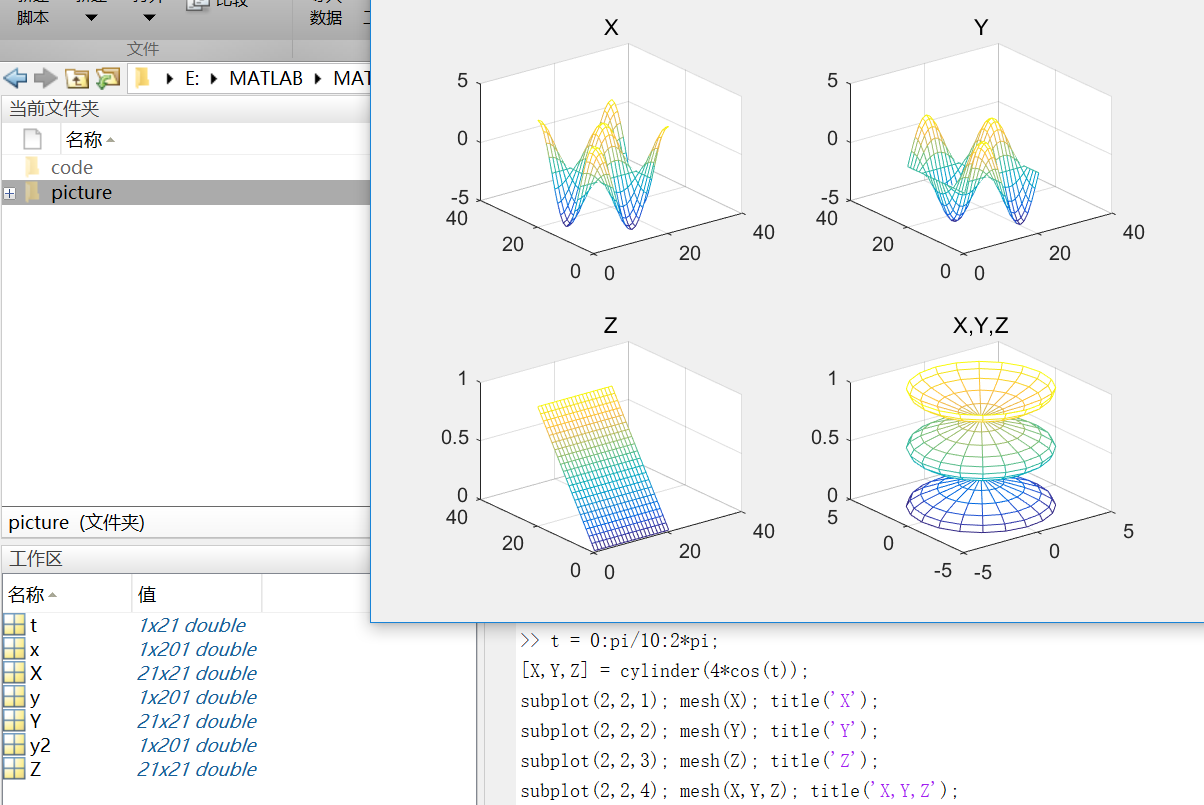mesh()生成由X，Y和Z指定的网线面，由C指定的颜色的三维网格图。
第八部分：程序和脚本
https://cn.mathworks.com/help/matlab/learn_matlab/scripts.html
示例脚本（从均匀分布中生成随机数据并计算平均值。绘制数据和平均值。）  用edit命令来创建脚本：  edit plotrand  这样就打开了一个叫plotrand.m的空文件，输入产生随机数向量的代码：
n = 50;
r = rand(n,1);
plot(r)

然后，添加代码在图中绘制水平线：
m = mean(r);
hold on
plot([0,n],[m,m])
hold off
title('Mean of Random Uniform Data')

在写代码过程中，可用（%）在代码之间添加注释。  最后保存脚本，在命令行窗口输入plotrand运行脚本。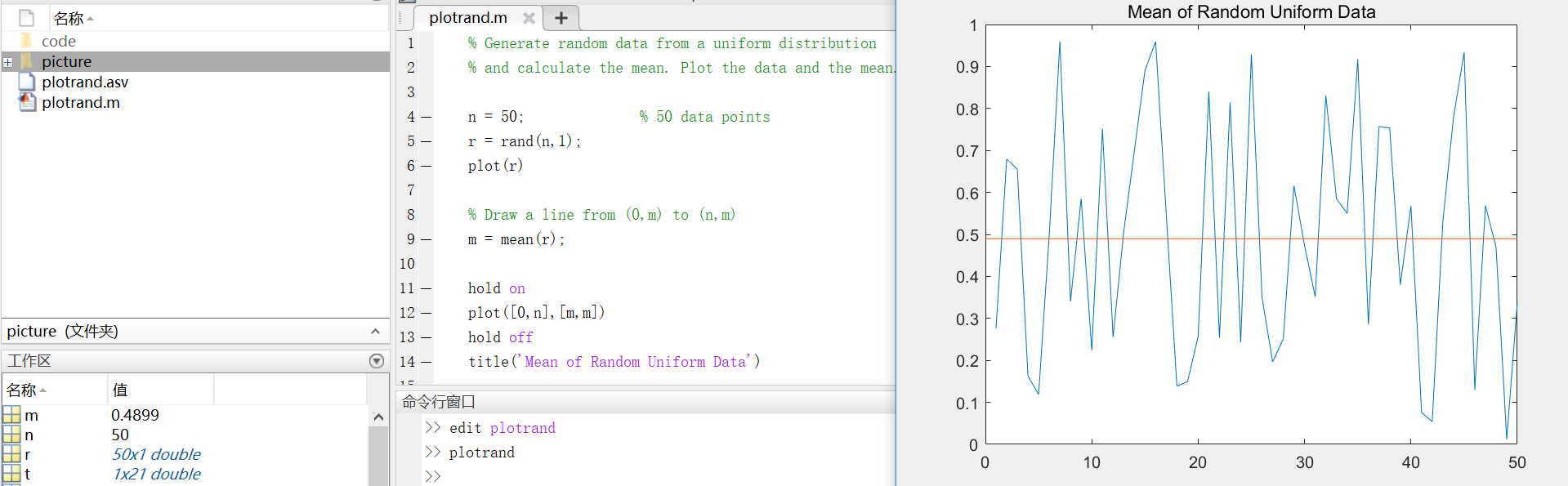循环和条件语句  在脚本中，可以循环使用部分代码，并且通过使用（for,while,if,switch等关键字）来执行这部分代码。  例如，创建一个叫calcmean.m的脚本，利用for循环计算五个随机样本的平均数和总平均数。
nsamples = 5;
npoints = 50;

for k = 1:nsamples
currentData = rand(npoints,1);
sampleMean(k) = mean(currentData);
end
overallMean = mean(sampleMean)
现在，修改for循环，以便每次迭代时都可以查看结果。在运行脚本时，会先显示中间结果，再计算总体均值。
for k = 1:nsamples
iterationString = ['Iteration #',int2str(k)];
disp(iterationString)
currentData = rand(npoints,1);
sampleMean(k) = mean(currentData)
end
overallMean = mean(sampleMean)

calcmean
在编辑器中，在calcmean.m的最后添加条件语句，使它根据不同的总体均值返回不同的信息。
if overallMean < .49
disp('Mean is less than expected')
elseif overallMean > .51
disp('Mean is greater than expected')
else
disp('Mean is within the expected range')
end
脚本位置  MATLAB中，要运行脚本，文件必须位于当前文件夹或搜索路径中的文件夹中。  默认情况下，MATLAB安装程序创建的MATLAB文件夹位于搜索路径上。如果要在另一个文件夹中存储和运行程序，需要将其添加到搜索路径中：在当前文件夹浏览器中选择文件夹，右键单击，然后选择“添加到路径”。
第九部分：帮助和文档
https://cn.mathworks.com/help/matlab/learn_matlab/help.html
使用doc命令在单独的窗口中打开函数文档。 doc mean键入左圆括号后暂停，MATLAB在命令窗口中就会显示函数提示（函数文档的语法部分）。 mean(使用帮助命令后，在命令窗口中查看函数的简单用法概括。 help mean
以上就是MATLAB的基础入门知识，欢迎指正呦~
展开全文• 最大合成法：最大合成，就是将来多幅相同的栅格图叠加，每个栅格单元取多幅中最大的那个，最后合成一幅。 归一化植被指数【NDVI=(NIR-R)/(NIR+R)】 1、检测植被生长状态、植被覆盖度和消除部分辐射误差。 2、-1<...
​教程照片及其他详细信息请关注微信公众号：夫也的笔记 公众号内容包含：ArcGIS、ENVI、MATLAB、Python和R语言教程和实际案例分享
理论知识 最大合成法：最大合成，就是将来多幅相同的栅格图叠加，每个栅格单元值取多幅中最大的那个，最后合成一幅。 归一化植被指数【NDVI=(NIR-R)/(NIR+R)】 1、检测植被生长状态、植被覆盖度和消除部分辐射误差。 2、-1<=NDVI<=1，负值表示地面覆盖为云、水、雪等，对可见光高反射；0表示有岩石或裸土等，NIR和R近似相等；正值，表示有植被覆盖，且随覆盖度增大而增大； 3、NDVI的局限性表现在，用非线性拉伸的方式增强了NIR和R的反射率的对比度。对于同一幅图象，分别求RVI和NDVI时会发现，RVI值增加的速度高于NDVI增加速度，即NDVI对高植被区具有较低的灵敏度。 4、NDVI能反映出植物冠层的背景影响，如土壤、潮湿地面、雪、枯叶、粗糙度等，且与植被覆盖有关。 NDVI的获取一方面，可以在NASA的官方网站上直接下载成品数据，数据的分辨率分别为250米、500米、1000米，根据应用目的的不同用户自行选择。另一方面，可以下载遥感影像，根据NDVI=(NIR-R)/(NIR+R)进行波段运算，不过这对遥感影像的质量要求比较高，需要影像上的云量比较少，必要的话还需要进行去云处理。 NDVI（近红外区与红光区的反射率差值/近红外区与红光区的反射率和值）是最常用的植被指数，虽然NDVI对土壤背景的变化较为敏感，但由于NDVI可以消除大部分与仪器定标、太阳角、地形、云阴影和大气条件有关辐照度的变化，增强了对植被的响应能力，是目前已有的40多种植被指数中应用最广的一种。
采用最大合成法将月数据NDVI合成年数据NDVI，本文以SPOT的月NDVI为例进行，利用Matlab平台，代码如下所示： %author [a,R]=geotiffread(‘F:\项目\data\spot_月ndvi\ndvi200001.tif’);%先投影信息 info=geotiffinfo(‘F:\项目\data\spot_月ndvi\ndvi200001.tif’); for i=2000:2015 datasum=zeros(size(a,1)*size(a,2),12); %size(a,1)和size(a,2)分别是进行合成的图像的行列号 for j=1:12 if j<10 data=importdata(strcat(‘F:\项目\data\spot_月ndvi\ndvi’,int2str(i),‘0’,int2str(j),’.tif’)); else data=importdata(strcat(‘F:\项目\data\spot_月ndvi\ndvi’,int2str(i),int2str(j),’.tif’)); end data=reshape(data,size(a,1)*size(a,2),1); datasum(:,j)=data; end maxndvi=zeros(size(a,1),size(a,2))+NaN; for n=1:length(datasum) data=datasum(n,:); datamax=max(data); if datamax>-1 maxndvi(n)=datamax; end end filenameet=strcat(‘F:\项目\data\2000-2015年NDVI\spot_ndvi_’,int2str(i),’.tif’); geotiffwrite(filenameet,maxndvi,R,‘GeoKeyDirectoryTag’,info.GeoTIFFTags.GeoKeyDirectoryTag); end
欢迎关注、转发、点赞、打赏
展开全文• ## matlab算术运算

千次阅读 2019-11-06 14:51:50
MATLAB算术运算 MATLAB的算术运算有两种不同类型：1）矩阵算术运算；2）阵列算术运算MATLAB矩阵算术运算与线性代数中的定义相同：执行数组操作，无论是在一维和多维数组元素的元素。 矩阵运算符和数组运营商是...
MATLAB算术运算
MATLAB的算术运算有两种不同类型：1）矩阵算术运算；2）阵列算术运算。
MATLAB矩阵算术运算与线性代数中的定义相同：执行数组操作，无论是在一维和多维数组元素的元素。
矩阵运算符和数组运营商是有区别的句点（.）符号。然而，由于加法和减法运算矩阵和阵列是相同的，操作者这两种情况下是相同的。
下表给出了运算符的简要说明：
操作符描述+加法或一元加号。A + B将A和B。 A和B必须具有相同的尺寸，除非一个人是一个标量。一个标量，可以被添加到任何大小的矩阵。-减法或一元减号。A - B，减去B从A和B必须具有相同的大小，除非是一个标量。可以从任意大小的矩阵中减去一个标量。*   矩阵乘法；是一个更精确的矩阵A和B的线性代数积， 矩阵乘法对于非纯量A和B，列一个数必须等于B.标量可以乘以一个任意大小的矩阵的行数。   .*数组的乘法；A.*B是数组A和B的元素积，A和B必须具有相同的大小，除非A、B中有一个是标量。/ 斜线或矩阵右除法；B/A与B * inv（A）大致相同。更确切地说：  B/A = (A'B')' ./矩阵右除法；矩阵A与矩阵B相应元素相除（A、B为同纬度的矩阵）.\反斜杠或矩阵左除；如果A是一个方阵，AB是大致相同的INV（A）* B，除非它是以不同的方式计算。如果A是一个n*n的矩阵，B是一个n组成的列向量，或是由若干这样的列的矩阵，则X = AB 是方程 AX = B ，如果A严重缩小或者几乎为单数，则显示警告消息。.数组左除法；A. B是元素B（i，j）/A（i，j）的矩阵。A和B必须具有相同的大小，除非其中一个是标量。^矩阵的幂。X^P是X到幂P，如果p是标量；如果p是一个整数，则通过重复平方计算功率。如果整数为负数，X首先反转。对P值的计算，涉及到特征值和特征向量，即如果[ D ] = V，EIG（x），那么X^P = V * D.^P / V。.^A.^B：A的每个元素的B次幂（A、B为同纬度的矩阵）'矩阵的转置；A'是复数矩阵A的线性代数转置，这是复共轭转置。.'数组的转置；A'是数组A的转置，对于复数矩阵，这不涉及共轭。
MATLAB算术运算功能
除了上述列举的一些算术运算符，MATLAB 中还拥有以下的命令/功能：
函数描述uplus(a)一元加号；增加量aplus (a,b)相加；返回 a + buminus(a)一元减号；减少aminus(a, b)相减；返回 a - btimes(a, b)数组相乘；返回 a.*bmtimes(a, b)矩阵相乘；返回 a* brdivide(a, b)右阵划分；返回 a ./ bldivide(a, b)左阵划分；返回 a. bmrdivide(A, B)求解线性方程组xA = B for xmldivide(A, B)求解线性方程组xA = B for xpower(a, b)数组求幂；返回 a.^bmpower(a, b)矩阵求幂；返回 a ^ bcumprod(A) 累积乘积；返回与包含累积乘积的数组A相同大小的数组。
如果A是向量，则cumprod（A）返回一个包含A的元素的累积乘积的向量。  如果A是矩阵，则cumprod（A）返回一个矩阵，其中包含A的每一列的累积乘积。  如果A是一个多维数组，那么cumprod（A）将沿着第一个非正整数维。 cumprod(A, dim)沿维 dim 返回返回累积乘积。cumsum(A) 累加总和；返回包含累积和的数组A
如果A是向量，则cumsum（A）返回一个包含A的元素的累积和的向量。  如果A是矩阵，则cumsum（A）返回一个矩阵，其中包含A的每列的累积和。  如果A是一个多维数组，那么cumsum（A）将沿着第一个非整数维度起作用。 cumsum(A, dim)返回沿着dim的元素的累积和。diff(X) 差分和近似导数；计算x相邻元素之间的差异。
如果X是向量，则diff（X）返回相邻元素之间的差异的向量，比X短一个元素：[X（2）-X（1）X（3）-X（2）... X（N）-X（N-1）]  如果X是一个矩阵，则diff（X）返回行差的矩阵：[X（2：m，...）-X（1：m-1，:)] diff(X,n)递归应用n次，导致第n个差异。diff(X,n,dim)它是沿标量dim指定的维数计算的第n个差分函数。 如果order n等于或超过Dim的长度，diff将返回一个空数组。prod(A) 数组元素的乘积；返回A数组元素的乘积。
如果A是向量，则prod（A）返回元素的乘积。  如果A是非空矩阵，则prod（A）将A的列作为向量，并返回每列乘积的行向量。  如果A是一个空的0-by-0矩阵，则prod（A）返回1。  如果A是一个多维数组，那么prod（A）将沿着第一个非子集维度行为并返回一个乘积数组。 该维数的尺寸减小到1，而所有其他维数的尺寸保持不变。 如果输入A为单个，则prod函数计算并返回B为单个；对于所有其他数字和逻辑数据类型，prod函数计算并返回B为double。 prod(A,dim)沿dim维度返回乘积。 例如，如果A是矩阵，则prod（A，2）是包含每一行的乘积的列向量。prod(___,datatype)在数据类型指定的类中乘以并返回一个数组。sum(A)
数组元素的总和；返回数组的不同维度的和。如果A是浮动的，那么是双倍或单个，B是本地累加的，它与A相同，B与A具有相同的类。如果A不是浮动的，则B被累加为双，B具有类double。  如果A是向量，则sum（A）返回元素的总和。  如果A是矩阵，则sum（A）将A的列作为向量，返回每列的和的行向量。  如果A是一个多维数组，sum（A）将沿着第一个非单例维度的值作为向量来处理，返回一个行向量的数组。 sum(A,dim)沿标量A的维度求和。 sum(..., 'double') sum(..., dim,'double') 执行双精度加法，并返回double类型的答案，即使A具有数据类型单一或整型数据类型。这是整型数据类型的默认值。 sum(..., 'native') sum(..., dim,'native') 在本机数据类型A中执行添加，并返回相同数据类型的答案。 这是单和双的默认值。ceil(A)向正无穷方向舍入；将a元素舍入为大于或等于A的最近整数。fix(A)舍入为零floor(A)向负无穷方向舍入；将a元素舍入为小于或等于a的最近整数。 idivide(a, b) idivide(a, b,'fix') 整数除法的舍入选项；与A./B相同，只是分数的商向零舍入到最接近的整数。idivide(a, b, 'round')分数的商舍入到最近的整数。idivide(A, B, 'floor')分数商向负无穷大舍入到最接近的整数。idivide(A, B, 'ceil')分数商向无穷大舍入到最接近的整数。mod (X,Y) 除法后的模数；返回X - n.* Y，其中 n = floor（X./Y）。 如果Y不是整数，并且商X / Y在整数的舍入误差内，则n是整数。 输入X和Y必须是相同大小的真实数组或实数标量（提供Y〜= 0）。 请注意：
mod(X,0) 是 X  mod(X,X) 是 0  对于 X ＝ Y 和 Y ＝ 0的 mod(X，Y)具有与Y相同的符号。 rem (X,Y) 除法之后的余数；返回X - n.* Y，其中n = fix（X./Y）。 如果Y不是整数，并且商X / Y在整数的舍入误差内，则n是整数。 输入X和Y必须是相同大小的真实数组或实数标量（提供Y〜= 0）。 请记住：
rem(X,0) 是 NaN  X〜= 0的rem（X，X）为0  对于 X~=Y 和 Y~=0 的rem（X，Y）与X具有相同的符号。 round(X)舍入到最接近的整数; 将X的元素舍入到最接近的整数。 正数元素的小数部分为0.5，最大到最接近的正整数。 负数元素的小数部分为-0.5，向下舍入到最接近的负整数。
展开全文• ## OFDM完整仿真过程及解释（MATLAB）

万次阅读 多人点赞 2019-04-19 17:03:45
因为是复制过来，如果出现图片显示... OFDM完整仿真过程及解释（MATLAB） - 子木的文章 - 知乎 https://zhuanlan.zhihu.com/p/57967971 目录： 一、说明 二、ofdm总体概述 ...六、OFDM的MATLAB仿真程序 一、说...OFDM QPSK
• ## MATLAB实现彩色图像灰度化

千次阅读 多人点赞 2019-12-12 19:05:26
实现彩色图像的灰度化主要有三种方法：最大值法、平均值法、加权平均法。 最大值法 取RGB三色分量中的最大值，即R=G=B=max（R,G,B） 平均值法 取RGB三色分量中的平均值，即R=G=B=(R+G+B)/3 加权平均法 – ...
• ## matlab人脸识别论文

万次阅读 多人点赞 2019-10-11 17:41:51
前者指根据人脸定位结果将图像中的人脸变化到同一位置和大小:后者是指对图像进行光照补偿等处理，以克服光照变化的影响，光照补偿能够一定程度的克服光照变化的影响而提高识别率。提取出待识别的人脸特征之后，即...
• ## MATLAB01:基本的数学运算与矩阵运算

万次阅读 多人点赞 2019-11-12 17:22:52
MATLAB01:基本的数学运算与矩阵运算MATLAB基本语法变量变量名保留变量不适合做变量名变量不应当覆盖内置函数MATLAB的调用优先级变量类型数字型变量的显示格式MATLAB命令行使用MATLAB进行数字运算使用MATLAB计算数学...矩阵操作
• %振幅最大值 amp_min = min(fft_amp); %振幅最小值 amp_median = median(fft_amp); %振幅中位数 amp_mean = mean(fft_amp); %振幅平均值 amp_pk = amp_max - amp_min; %振幅峰差 amp_mph = amp_pk * 0.75; %振幅...信号特征 特征提取 时域
• ## Matlab遗传算法求函数最大值

万次阅读 多人点赞 2016-10-13 20:06:45
主函数函数main.mglobal Bitlength%定义3个全局变量 global boundsbegin global boundsend boundsbegin=-2;...%运算精确度 Bitlength=ceil(log2((boundsend-boundsbegin)'./precision));%染色体长度 popsize=50函数 算法 遗传算法 数学建模
• 基于MATLAB的直接序列扩频通信系统仿真 前言 文章迭代更新 仿真流程图 关键技术细节 扩频解扰解扩部分 加扰去扰部分 加扰原理图 调制解调部分 调制原理图 解调原理图 高斯信道部分 实验结果 扩频增益为10时，walsh...直接序列扩频系统
• ## Matlab绘图

千次阅读 2018-05-27 10:36:24
Matlab绘图 强大的绘图功能是Matlab的特点之一，Matlab提供了一系列的绘图函数，用户不需要过多的考虑绘图的细节，只需要给出一些基本参数就能得到所需图形，这类函数称为高层绘图函数。此外，Matlab还提供了直接对...
• ## 模糊PID算法及其MATLAB仿真（1）

万次阅读 多人点赞 2019-04-15 20:34:35
算数运算可以表示为： 另外还有最大最小运算、布尔运算、标准法运算等。 其实需要注意的主要就是模糊运算与常规的运算之间的区别。比如在模糊控制中看起来可能是交运算符（^），但是实际上是个取极小值运算。 4.5、...Fuzzy PID
• 对于较大数乘积运算结果中的inf，可以采用sym（a）符号变量解决。 例如，求区间[1，1000000]内所有质数的乘积。 若直接运行： tic A=1:1000000; B=A(isprime(A)); prod(B) toc 得出的结果将是inf，这样并不知道结果...inf
• ## 基于MATLAB的语音信号处理

万次阅读 多人点赞 2018-07-15 01:21:20
基于MATLAB的语音信号处理摘要：语音信号处理是...MATLAB软件以其强大的运算能力可以很好的完成对语音信号的处理。通过MATLAB可以对数字化的语音信号进行时频域分析，方便地展现语音信号的时域及频域曲线，并且根...
• ## 【MATLAB】MATLAB的基础知识

千次阅读 多人点赞 2017-04-12 11:52:00
MATLAB的启动和退出1.MATLAB的启动有如下两种方式： 方式一：双击操作系统桌面上的MATLAB快捷方式，即可启动并打开MATLAB命令窗口。 方式二：单击【开始】菜单，依次指向【程序】→【MATLAB】即可启动并打开...
• ## MATLAB矩阵运算函数

千次阅读 2020-08-09 15:52:29
向量无穷--范数：所有向量元素绝对值中的最大值 norm[V,inf]; 十一.矩阵的条件数 矩阵的条件数等于A的范数与A的逆矩阵的范数的乘积； 条件数越接近于1，矩阵的性能越好，反之，矩阵的性能越差。 cond[A,1]; cond[A]...
• matlab代码MSA：多微扰沙普利分析工具箱 作者：Shay Ofir-Geva 和 Isaac Meil​​ijson。 这是 Matlab 工具箱，实现了用于病变行为/症状映射 (LBM) 的多扰动 Shapley 分析。 目录 基本信息 多扰动 Shapley ...
• ## matlab多项式及其运算

万次阅读 多人点赞 2018-11-17 21:19:00
matlab中，提供了poly2sym函数实现多项式的构造。 r = poly2sym(c)：c为多项式的系数向量 r = poly2sym(c, v)：c为多项式的系数向量，v为其变量 &gt;&gt; poly2sym([1 3 2]) ans = x^2 + 3*x + 2 &...多项式 基础 语言 笔记
• 此函数将需要以下输入以计算预期结果： 1- Inputdata_signal：一个包含你需要的 Y 的向量过滤。 2- Inputdata_time：一个包含您需要的 x 的向量过滤（通常是一个时间信号，其中采样率可以提取）。 3- ...
• ## matlab常用逻辑运算

千次阅读 2018-04-23 13:01:48
ans最近计算的答案clc清除命令行窗口diary将命令行窗口文本保存到文件中format设置命令行窗口输出显示格式home发送光标复位iskeyword确定输入是否为 MATLAB 关键字more控制命令行窗口分页输出矩阵和数组zeros创建...
•线性代数 矩阵
• ## MATLAB控制运算精度

万次阅读 2014-08-04 10:36:51
先来看一段MATLAB代码  clear;  theta = 264.3783;  t1 = [cos(theta); cos(2*theta);cos(3*theta); cos(4*theta); sin(theta); sin(2*theta); sin(3*theta);sin(4*theta); 1];   t2 =[0.8849 0.5661 0...
• ## 蚁群算法---matlab代码

万次阅读 多人点赞 2017-08-03 00:39:50
蚁群算法—matlab代码文明转帖，代码摘自：FPGA机器学习之蚁群算法 matlab程序蚁群算法简介 蚁群是自然界中常见的一种生物，人们对蚂蚁的关注大都是因为“蚁群搬家，天要下雨”之类的民谚。然而随着近代仿生学的...机器学习 蚁群算法
• 遗传算法的运算对象是表示个体的符号串，所以必须把变量 x1, x2 编码为一种 符号串。本题中，用无符号二进制整数来表示。 因 x1, x2 为 0 ~ 7之间的整数，所以分别用3位无符号二进制整数来表示，将它 们连接在...
• OTSU算法是由日本学者OTSU于1979年提出的一种对图像进行二化的高效算法。（大津算法） Otsu原理 对于图像 t (x,y)，前景(即目标)和背景的分割阈值记作 T，属于前景的像素点数占整幅图像的比例记为 ω0，平均灰度为...数字图像处理 otsu算法
• 下面写如何用MATLAB具体实现上述过程。 clc;clear;close all; %% 初始化种群 f= @(a,b)(a .* sin(a) .* cos(2 * a) - 2 * a .* sin(3 * a)).*(b .* sin(b) .* cos(2 * b) - 2 * b .* sin(3 * b)); % 函数表达式 ...粒子群算法...

# matlab运算结果最大值matlab 订阅